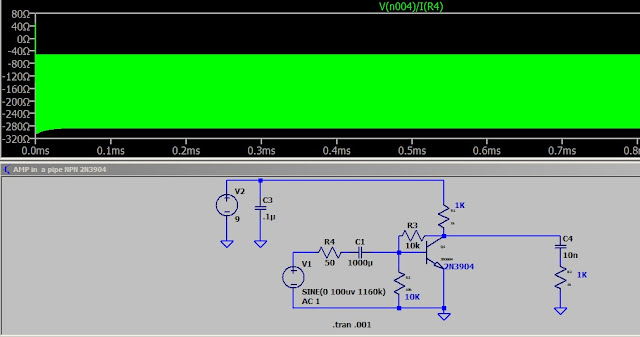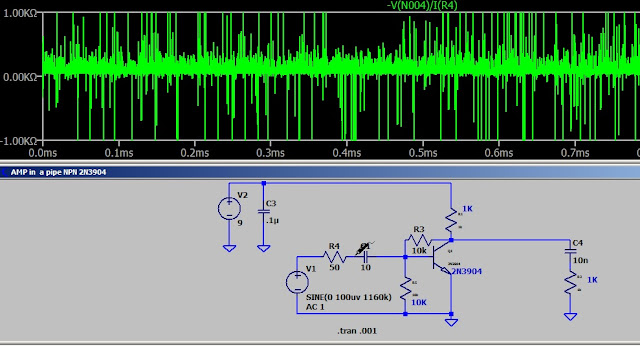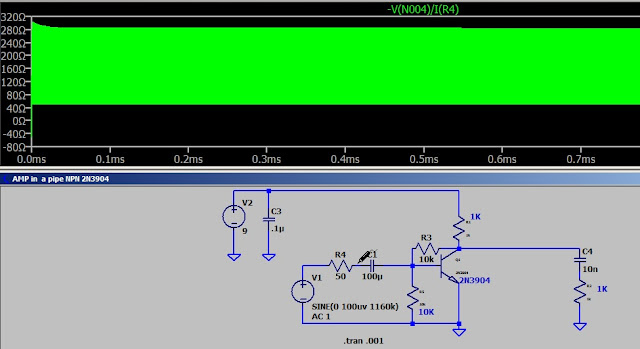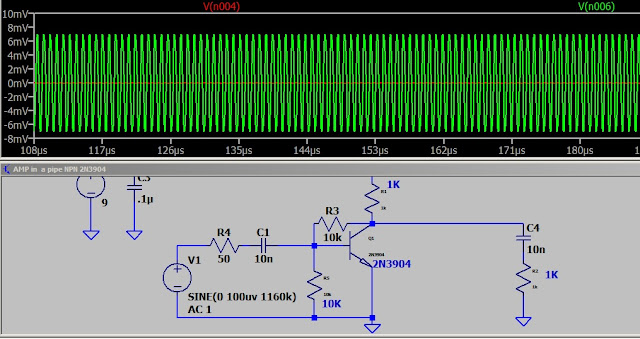## Monday, January 7, 2019

### amp in a pipe - self biasing examined

This is the basic idea. I have made a couple on a breadboard and they perform well. I think I could use 2 for preamps on my scope and make it more useful. The low range is in milli volts. With the amp I could read micro volts. That's the plan.
node 3 and 4 are either side of R4 and node 6 is the output. When I measure node 4 and divide by the current through R4 it give me the input Z. It is a dynamic reading so we have to take the average. 150 ohms ? Basically the base emitter junction and Xc1.
I have some surface mount 2N3904 for this one. The arrows point to nodes 3, 4 and 6. I'll be taking reading from these nodes to determine the impedance and gain.
It look good.
I measured node 4 and divided by I(R4) to get the input Z.
I cleaned up the chatter from the previous shot.The larger capacitor charges and holds the voltage to make it appear that we have a negative resistance. It is the phase shift through the capacitor.In this shot I moved the reference to one side of R4 and measured across it. The floating ground allowed noise to display but the 50 ohm resistor is showing.Here I leave the reference at R4 and measure to ground to get the input Z. When I did the same measurement with a 1000u cap the reading was negative. The 100u cap show a positive reading. The RC time constant is determining the reaction to frequency.Back to the original circuit.Here we see the 50 ohm generator ZIf I had Zgen = Zin I would read 50uv with the generator set at 100uv. How would I adjust that? Does it need adjusting?100uv in and 7000uv out. The Zin could be adjusted by changing Ic. This could be done by adjusting the base emitter resistor or the load resistor. Changing either one would vary Vc which in turn would change Vb and cause a shift in Ic. If you plot a load line you would see the circuit gain changes with slope. So making either resistor adjustable would allow the gain and Z to be varied.
Zin is low so this would be a good circuit for a 50 ohm system. It would load a high Z circuit. Why use 50 ohm? A good question that could use a good answer. Maybe a topic for another time.